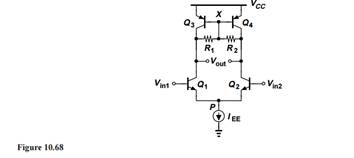### Create an Account

Home / Questions / Consider the circuit shown in Fig 1068 where EE 2 mA VA n 5 V VAp 4 V.What value of R1 ...

# Consider the circuit shown in Fig 1068 where EE 2 mA VA n 5 V VAp 4 V.What value of R1 R2 allows a voltage gain of 50

Consider the circuit shown in Fig. 10.68, whereEE = 2 mA, VA,n = 5 V VA,p = 4 V. What value of R1 = R2 allows a voltage gain of 50?Apr 24 2020 View more View LessSubscribe To Get Solution